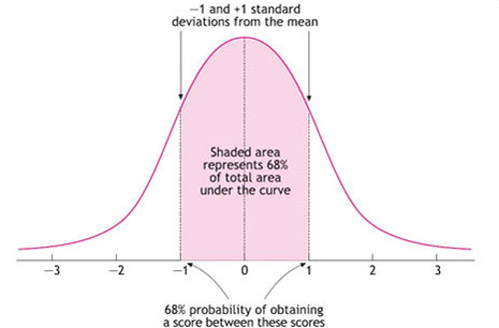Understanding dyslexia

Start this free course now. Just create an account and sign in. Enrol and complete the course for a free statement of participation or digital badge if available.

1.2.1 Statistical approaches to ‘normality’

What did you base your idea of ‘normal’ height on? It might have been based on your own experience, reflecting the average height of women in your community. Similarly, ‘abnormality’ can be defined in terms of low statistical frequency. If what is most common in the general population is considered ‘normal’, then any behaviour or psychological characteristic that occurs only rarely may be regarded as ‘abnormal’. From this viewpoint, above average individuals are just as ‘abnormal’ as those who are below average. This approach is particularly suited to variables that are dimensional, such as height or scores on a test. These variables lend themselves to measurement on a continuous scale. You can relate this to what you have already learned about the normal distribution. If you look at Figure 1, you can see the normal distribution curve with the mean value in the middle.

About 68 per cent of all values are within one standard deviation of the mean (Dancey and Reidy, 2002). This is termed the ‘normal’ zone of the curve. Note that this choice of the percentage of values which comes under the ‘normal’ zone of the curve is arbitrary in the sense that it was defined by statisticians, and does not reflect any natural circumstance or law.Figure 1 The normal distribution curve (Source: Dancey and Reidy, 2002, p. 89)

The standard deviation for a set of scores can therefore be used to define the boundaries of what ‘normal’ might be. Another example is that of IQ scores, which are constructed to be normally distributed with a mean of 100 and a standard deviation of 15. This means that IQ scores between 85 and 115 are considered to be ‘normal’. Scores lower than 85 are regarded as abnormally low (the bottom 16 per cent), and scores higher than 115 are seen as abnormally high (the top 16 per cent).

This seemingly simple and objective approach to defining ‘normality’ is in fact quite problematic. If deviation from statistical norms is used to define ‘abnormal’ functioning, then the following questions about measurement must be answered.

1. How are psychological characteristics (such as intelligence) quantified? Can they be measured on a continuous scale or are they better captured by discrete categories?

2. Do the relevant measures have a normal distribution? If not, mean scores and standard deviations are likely to be inappropriate reference points (Dancey and Reidy, 2002).

3. Are the assessments reliable? Tests that are going to be used for diagnostic purposes need to have extremely good internal and external reliability.

4. Are the assessments valid? To address this, we need to be clear about the purpose of measurement and consider different types of validity.

5. How is a behaviour classified as ‘abnormal’? The ‘one standard deviation away from the mean’ criterion can be a rather over-inclusive indicator of abnormality for some tasks, especially if the consequences of such an assessment would be to give that person a negative label. More extreme boundaries of 1.5 or 2 standard deviations from the mean are sometimes chosen instead. Any cut-off point for classifying people into discrete categories of ‘normal’ and ‘abnormal’ is arbitrary if the characteristic in question really is dimensional.

6. Does it matter if someone is scoring higher than the average population? On some measures very high scores may be just as worrying as very low scores (e.g. persistent over-arousal, reflecting stress or anxiety). On other measures, only one extreme of the distribution may be considered ‘abnormal’ in the sense of problematic. The statistical approach to defining normality does not make these kinds of judgements, so clearly other criteria are being applied in these situations.